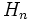# Sub-APS of groups

Let$(G,\Phi)$ be an APS of groups. A sub-APS$H$ of$G$ is, for every$n$, a subgroup$H_n$ of$G_n$ such that$\Phi_{m,n}(g,h) \in H_{m+n}$ whenever$g \in H_m, h \in H_n$. Thus,$H$ can be viewed as an APS of groups, in its own right.